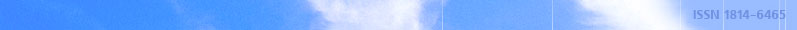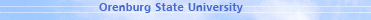december 07, 2021 RU/EN
 Headings of Vestnik Pedagogics Psychology Other

 Search Author Article

# April 2015, № 4 (179)

Shevelenko V.D., Lukoyanov V.A. USING OF THE ABEL TRANSFORM TO SUM TRIGONOMETRIC POLYNOMIALSCurrently, the spectral signal analysis commonly used digital filter based on DSP with the input of ADC (analog-to-digital converter), which implements the ordinary or fast discrete Fourier transform. Speed digital filter determines the width of the analyzed spectrum of the measured process and the ability to process data in real time. Most characteristic for the speed of digital filters based on dedicated processors fast Fourier transform, multiplying the matrix comprising a conveyor with parallel data processing. However, parallelization of computing operations leads to a nonlinear increase in the volume of equipment. Therefore, despite the rather significant process in improving the performance of digital element base, the computing speed of the need to perform parallel-serial number of multiplications is relatively increased substantially less, which leads to inefficient use of components. The upper cutoff frequency spectrum of the signal that can be analyzed not exceed a few dozen kilohertz, at a number of points 1024, and accordingly, the number of harmonics equal to 512. In solving the problems of filtration, requiring a large number of multiplications, to increase the speed of signal processing by the discrete Fourier transform is needed transition to digital-to-analog and analog multiplier with a maximum parallelization of the encoding and signal processing, and implementation of these operations as much as possible in the devices. This need is caused by the fact that the expansion of the frequency spectrum of the analyzed dimensions, current consumption and the cost of digital "Fourier-processor" increases dramatically.The article discusses the possibility of using the Abel transformation to summation of trigonometric functions. It shows some of the common sum of the coefficients. It considers conclusions, under which it is possible to exist special cases, when the using of second Abel transformation form to summation of trigonometric functions will be more appropriate.Key words: Abel transform, summability of series, Parseval equality, trigonometric functions, energy and information properties of the series sums.

References:

1. Digital signal processors based on BIS. / Makarevich OB, Spiridonov BG .// International electronics. 1983. №1., Pp 3-24 .;

2. Gelman, MM System analog-to-digital converters and signal processors. Gelman MM M: Mir, 1999, 381 p .;

3. Baron, S. Introduction to the theory of series summability / Baron S. — Tallin: Valgus, 1977., 280 p.

4. Abel transform as a tool for implementing of the orthonormal basis filtering properties / Schurin V.B., Shevelenko V.D., Schurin K.V., Lukoyanov V.A.// Collected materials of the International scientific-practical conference "Modern materials, equipment and technology" ., 22 december 2011., Kursk, 2011. P. 356-360.Editor-in-chief Sergey Aleksandrovich MIROSHNIKOV